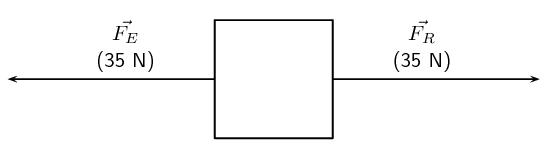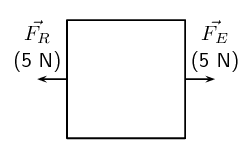Home Practice
For learners and parents For teachers and schools
Textbooks
Full catalogue
Pricing SupportLog in

We think you are located in United States. Is this correct?

# 20.3 Properties of vectors

## 20.3 Properties of vectors (ESAGN)

Vectors are mathematical objects and we will now study some of their mathematical properties.

If two vectors have the same magnitude (size) and the same direction, then we call them equal to each other. For example, if we have two forces, $$\vec{F_{1}} = \text{20}\text{ N}$$ in the upward direction and $$\vec{F_{2}} = \text{20}\text{ N}$$ in the upward direction, then we can say that $$\vec{F_{1}} = \vec{F_{2}}$$.

Equality of vectors

Two vectors are equal if they have the same magnitude and the same direction.

Just like scalars which can have positive or negative values, vectors can also be positive or negative. A negative vector is a vector which points in the direction opposite to the reference positive direction. For example, if in a particular situation, we define the upward direction as the reference positive direction, then a force $$\vec{F_{1}} = \text{30}\text{ N}$$ downwards would be a negative vector and could also be written as $$\vec{F_{1}} = -\text{30}\text{ N}$$. In this case, the negative sign ($$-$$) indicates that the direction of $$\vec{F_{1}}$$ is opposite to that of the reference positive direction.

Negative vector

A negative vector is a vector that has the opposite direction to the reference positive direction.

Like scalars, vectors can also be added and subtracted. We will investigate how to do this next.

temp text

### Addition and subtraction of vectors (ESAGO)

When vectors are added, we need to take into account both their magnitudes and directions.

For example, imagine the following. You and a friend are trying to move a heavy box. You stand behind it and push forwards with a force $$\vec{{F}_{1}}$$ and your friend stands in front and pulls it towards them with a force $$\vec{{F}_{2}}$$. The two forces are in the same direction (i.e. forwards) and so the total force acting on the box is: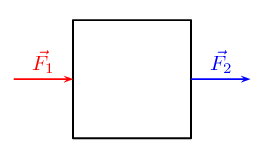It is very easy to understand the concept of vector addition through an activity using the displacement vector.

Displacement is the vector which describes the change in an object's position. It is a vector that points from the initial position to the final position.

### Method

Tape a line of masking tape horizontally across the floor. This will be your starting point.

Take $$\text{2}$$ steps in the forward direction. Use a piece of masking tape to mark your end point and label it A. Then take another $$\text{3}$$ steps in the forward direction. Use masking tape to mark your final position as B. Make sure you try to keep your steps all the same length!

Go back to your starting line. Now take $$\text{3}$$ steps forward. Use a piece of masking tape to mark your end point and label it B. Then take another $$\text{2}$$ steps forward and use a new piece of masking tape to mark your final position as A.

### Discussion

What do you notice?

1. In Task 1, the first $$\text{2}$$ steps forward represent a displacement vector and the second $$\text{3}$$ steps forward also form a displacement vector. If we did not stop after the first $$\text{2}$$ steps, we would have taken $$\text{5}$$ steps in the forward direction in total. Therefore, if we add the displacement vectors for $$\text{2}$$ steps and $$\text{3}$$ steps, we should get a total of $$\text{5}$$ steps in the forward direction.

2. It does not matter whether you take $$\text{3}$$ steps forward and then $$\text{2}$$ steps forward, or two steps followed by another $$\text{3}$$ steps forward. Your final position is the same! The order of the addition does not matter!

We can represent vector addition graphically, based on the activity above. Draw the vector for the first two steps forward, followed by the vector with the next three steps forward.

We add the second vector at the end of the first vector, since this is where we now are after the first vector has acted. The vector from the tail of the first vector (the starting point) to the head of the second vector (the end point) is then the sum of the vectors.

As you can convince yourself, the order in which you add vectors does not matter. In the example above, if you decided to first go $$\text{3}$$ steps forward and then another $$\text{2}$$ steps forward, the end result would still be $$\text{5}$$ steps forward.

#### Subtracting vectors

Let's go back to the problem of the heavy box that you and your friend are trying to move. If you didn't communicate properly first, you both might think that you should pull in your own directions! Imagine you stand behind the box and pull it towards you with a force $$\vec{{F}_{1}}$$ and your friend stands at the front of the box and pulls it towards them with a force $$\vec{{F}_{2}}$$. In this case the two forces are in opposite directions. If we define the direction your friend is pulling in as positive then the force you are exerting must be negative since it is in the opposite direction. We can write the total force exerted on the box as the sum of the individual forces: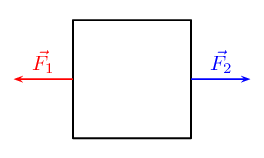What you have done here is actually to subtract two vectors! This is the same as adding two vectors which have opposite directions.

As we did before, we can illustrate vector subtraction nicely using displacement vectors. If you take $$\text{5}$$ steps forward and then subtract $$\text{3}$$ steps forward you are left with only two steps forward: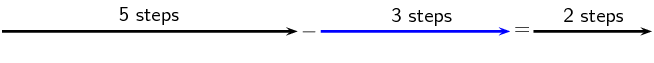What did you physically do to subtract $$\text{3}$$ steps? You originally took $$\text{5}$$ steps forward but then you took $$\text{3}$$ steps backward to land up back with only $$\text{2}$$ steps forward. That backward displacement is represented by an arrow pointing to the left (backwards) with length $$\text{3}$$. The net result of adding these two vectors is $$\text{2}$$ steps forward: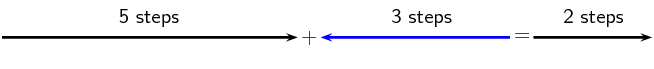Thus, subtracting a vector from another is the same as adding a vector in the opposite direction (i.e. subtracting $$\text{3}$$ steps forwards is the same as adding $$\text{3}$$ steps backwards).

Subtracting a vector from another is the same as adding a vector in the opposite direction.

#### The resultant vector

The final quantity you get when adding or subtracting vectors is called the resultant vector. In other words, the individual vectors can be replaced by the resultant – the overall effect is the same.

Resultant vector

The resultant vector is the single vector whose effect is the same as the individual vectors acting together.

We can illustrate the concept of the resultant vector by considering our two situations in using forces to move the heavy box. In the first case (on the left), you and your friend are applying forces in the same direction. The resultant force will be the sum of your two applied forces in that direction. In the second case (on the right), the forces are applied in opposite directions. The resultant vector will again be the sum of your two applied forces, however after choosing a positive direction, one force will be positive and the other will be negative and the sign of the resultant force will just depend on which direction you chose as positive. For clarity look at the diagrams below.

Forces are applied in the same direction

(positive direction to the right)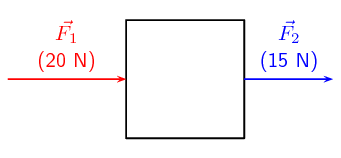Forces are applied in opposite directions

(positive direction to the right)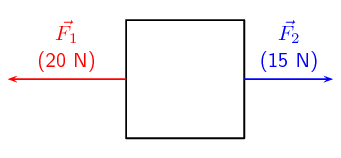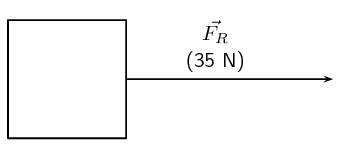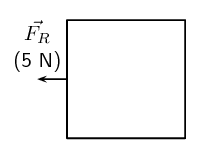There is a special name for the vector which has the same magnitude as the resultant vector but the opposite direction: the equilibrant. If you add the resultant vector and the equilibrant vectors together, the answer is always zero because the equilibrant cancels the resultant out.

Equilibrant

The equilibrant is the vector which has the same magnitude but opposite direction to the resultant vector.

If you refer to the pictures of the heavy box before, the equilibrant forces for the two situations would look like: